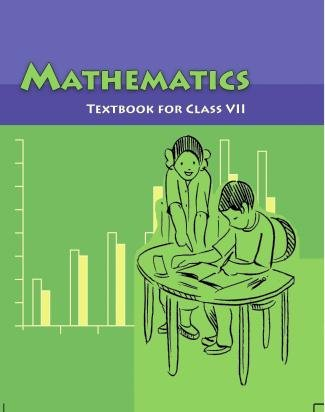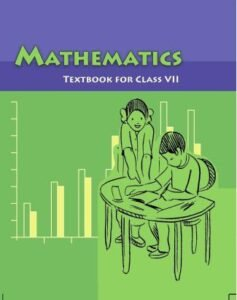NCERT Class 7 Maths Textbook PDF Download. CBSE has prescribed Class 7 Maths TextBook PDF. All schools affiliated to Central Board of Secondary Education follow the same NCERT Maths Class 7 Textbook PDF. Getting high score in VII Maths Exams is possible when student reads, understand and learn all concepts from NCERT book for Class 7 Maths.#### NCERT Class 7 Maths Book in Hindi Medium

You can download the NCERT Solutions For Class 7 Maths for Chapter 1 Integers , Chapter 2 Fractions And Decimals , Chapter 3 Data Handling , Chapter 4 Simple Equations , Chapter 5 Lines And Angles , Chapter 6 The Triangle And Its Properties , Chapter 7 Congruence Of Triangles , Chapter 8 Comparing Quantities , Chapter 9 Rational Numbers , Chapter 10 Practical Geometry , Chapter 11 , Perimeter And Area  , Chapter 12 Algebraic Expressions , Chapter 13 Exponents And Powers , Chapter 14 Symmetry , Chapter 15 Visualising Solid Shapes contain solved questions answers in PDF for free download based on latest guide / new CBSE Syllabus.

• Chapter 1 Integers
1.1 Introduction
1.2 Recall
1.3 Properties Of Addition And Subtraction Of Integers
1.4 Multiplication Of Integers
1.5 Properties Of Multiplication Of Integers
1.6 Division Of Integers
1.7 Properties Of Division Of Integers
• Chapter 2 Fractions And Decimals
2.1 Introduction
2.2 How Well Have You Learnt About Fractions?
2.3 Multiplication Of Fractions
2.4 Division Of Fractions
2.5 How Well Have You Learnt About Decimal Numbers
2.6 Multiplication Of Decimal Numbers
2.7 Division Of Decimal Numbers
• Chapter 3 Data Handling NCERT Solutions For Class 7 Maths
3.1 Introduction
3.2 Collecting Data
3.3 Organisation Of Data
3.4 Representative Values
3.5 Arithmetic Mean
3.6 Mode
3.7 Median
3.8 Use Of Bar Graphs With A Different Purpose
3.9 Chance And Probability
• Chapter 4 Simple Equations
4.2 Setting Up Of An Equation.
4.3 Review Of What We Know.
4.4 What Equation Is?
4.5 More Equations.
4.6 From Solution To Equation.
4.7 Applications Of Simple Equations To Practical Situations.
• Chapter 5 Lines And Angles
5.1 Introduction.
5.2 Related Angles.
5.3 Pairs Of Lines.
5.4 Checking For Parallel Lines.

• Chapter 6 The Triangle And Its Properties
6.1 Introduction.
6.2 Medians Of A Triangle.
6.3 Altitudes Of A Triangle.
6.4 Exterior Angle Of A Triangle And Its Property.
6.5 Angle Sum Property Of A Triangle.
6.6 Two Special Triangles Equilateral And Isosceles.
6.7 Sum Of The Lengths Of Two Sides Of A Triangle.
6.8 Right-Angled Triangles And Pythagoras Property.
• Chapter 7 Congruence Of Triangles
7.1 Introduction.
7.2 Congruence Of Plane Figures.
7.3 Congruence Among Line Segments.
7.4 Congruence Of Angles.
7.5 Congruence Of Triangles.
7.6 Criteria For Congruence Of Triangles.
7.7 Congruence Among Right-Angled Triangles.
• Chapter 8 Comparing Quantities
8.1 Introduction.
8.2 Equivalent Ratios.
8.3 Percentage Another Way Of Comparing Quantities.
8.4 Use Of Percentages.
8.5 Prices Related To An Item Or Buying And Selling.
8.6 Charge Given On Borrowed Money Or Simple Interest.
• Chapter 9 Rational Numbers
9.1 Introduction
9.2 Need For Rational Numbers.
9.3 What Are Rational Numbers?
9.4 Positive And Negative Rational Numbers.
9.5 Rational Numbers On A Number Line.
9.6 Rational Numbers In Standard Form.
9.7 Comparison Of Rational Numbers.
9.8 Rational Numbers Between Two Rational Number.
9.9 Operations On Rational Numbers.

• Chapter 10 Practical Geometry
10.1 Introduction
10.2 Construction Of A Line Parallel To A Given Line, Through A Point Not On The Line.
10.3 Construction Of Triangles.
10.4 Constructing A Triangle When The Lengths Of Its Three Sides Are Known (SSS Criterion)
10.5 Constructing A Triangle When The Lengths Of Two Sides And The Measure Of The Angle Between Them Are Known. (SAS Criterion)
10.6 Constructing A Triangle When The Measures Of Two Of Its Angles And The Length Of The Side Included Between Them Is Given. (ASA Criterion)
10.7 Constructing A Right-Angled Triangle When The Length Of One Leg And Its Hypotenuse Are Given (RHS Criterion).
• Chapter 11 Perimeter And Area
11.1 Introduction.
11.2 Squares And Rectangles.
11.3 Area Of A Parallelogram.
11.4 Area Of A Triangle.
11.5 Circles.
11.6 Conversion Of Units.
11.7 Applications.
• Chapter 12 Algebraic Expressions
12.1 Introduction.
12.2 How Are Expressions Formed?
12.3 Terms Of An Expression.
12.4 Like And Unlike Terms.
12.5 Monomials, Binomials, Trinomials And Polynomials.
12.6 Addition And Subtraction Of Algebraic Expressions.
12.7 Finding The Value Of An Expression.
12.8 Using Algebraic Expressions Formulas And Rules.
• Chapter 13 Exponents And Powers
13.1 Introduction.
13.2 Exponents.
13.3 Laws Of Exponents.
13.4 Miscellaneous Examples Using The Laws Of Exponents.
13.5 Decimal Number System.
13.6 Expressing Large Numbers In The Standard Form.
14. Symmetry.
• Chapter 14 Symmetry
14.2 Lines Of Symmetry For Regular Polygons.
14.3 Rotational Symmetry.
14.4 Line Symmetry And Rotational Symmetry.
• Chapter 15 Visualising Solid Shapes
15.1 Introduction Plane Figures And Solid Shapes.
15.2 Faces, Edges And Vertices.
15.3 Nets For Building 3-D Shapes.
15.4 Drawing Solids On A Flat Surface.
15.5 Viewing Different Sections Of A Solid.

## NCERT Class 7 Maths TextBook PDF

1. What is NCERT Maths Class 7 Textbook?

• The NCERT Maths Class 7 Textbook is a mathematics textbook designed for students in the 7th grade in India. It covers a wide range of mathematical concepts and topics.

• Yes, you can download the NCERT Maths Class 7 Textbook in PDF format for free from various educational websites and platforms. Simply search for “NCERT Maths Class 7 Textbook PDF download” to find it.

3. Why are NCERT Maths Class 7 Textbooks important?

• NCERT Maths Class 7 Textbooks are crucial because they provide a strong foundation in mathematics, which is essential for academic success and future studies in science and mathematics.

4. Are NCERT Maths Class 7 Textbooks suitable for self-study?

• Yes, NCERT Maths Class 7 Textbooks are designed to be user-friendly and can be used effectively for self-study. Many students use them as their primary resource for independent learning.

#### 5. Can I find specific chapters or topics from NCERT Maths Class 7 Textbook online?

• Yes, you can find individual chapters or topics from the NCERT Maths Class 7 Textbook online through educational websites and resources. Use relevant keywords to search for the specific topic you’re interested in.

6. Are there supplementary resources available along with NCERT Maths Class 7 Textbooks?

• NCERT provides supplementary resources like exercise solutions and exemplar problems to help students practice and deepen their understanding of mathematics.

7. Can students from all Indian states use NCERT Maths Class 7 Textbooks?

• Yes, NCERT books are widely accepted across India and are suitable for students in most states. However, some states may have their own educational boards with different textbooks, so it’s essential to check the local curriculum.

8. How can NCERT Maths Class 7 Textbooks help improve math skills?

• NCERT Maths Class 7 Textbooks offer clear explanations, diagrams, and exercises that help students understand and apply mathematical concepts. Regular practice with these books can significantly enhance math skills.

9. Are there online study materials and videos available to complement NCERT Maths Class 7 Textbooks?

• Yes, there are many online study materials, video lectures, and tutorials available that can complement your study with NCERT Maths Class 7 Textbooks. These resources provide additional explanations and visual aids to enhance the learning experience.

These frequently asked questions should help you understand the significance and accessibility of NCERT Maths Class 7 Textbooks and assist you in using them effectively for your mathematics studies and exam preparations.